Definitions of Square Dance Calls and Concepts

Triple formations Working direction \$B\$O(B, \$B\$\$\$/\$D\$+\$N(B 12-Matrix \$B%3!<%k\$r\$I\$N\$h\$&\$K9T\$&\$+\$K\$D\$\$\$F(B, \$B@53N\$K;X<(\$9\$k\$?\$a\$NJ}K!\$G\$9(B.

\$B\$3\$N%3%s%;%W%H\$O(B, Triple Boxes | Columns | Diamonds | Lines | Waves \$B\$J\$I\$N(B Triple formations \$B\$+\$i(B, 8 \$B?M\$N%3!<%k\$r9T\$(\$k\$h\$&\$K\$9\$k\$b\$N\$G\$9(B. \$BIaDL(B, \$B\$3\$l\$i\$N(B formation \$B\$G\$O(B 4 \$B?M(B (\$B\$^\$?\$O\$=\$l\$h\$j>/\$J\$\$?M(B) \$B\$N%3!<%k\$K8B\$i\$l\$^\$9(B. \$B\$=\$NM}M3\$O(B, \$B3F!9\$N(B sub-formation (Box, Column, Diamond, Line, \$B\$^\$?\$O(B Wave) \$B\$K\$O(B, 4 \$B\$D\$@\$1\$N>l=j\$,\$"\$k\$+\$i\$G\$9(B.

Triple formations Working direction \$B\$G\$O(B, \$B3F!9\$N?M\$O(B 12 \$B\$N>l=j\$+\$i(B, \$BF0\$-\$r9T\$&(B 8 \$B\$D\$N>l=j\$rA*\$S\$^\$9(B. \$B30B&\$N(B Triple formation \$B\$N?M\$O(B, \$B<+J,\$N(B Triple formation \$B\$N(B 4 \$B\$D\$N>l=j\$H(B, center Triple formation \$B\$N(B 4 \$B\$D\$N>l=j\$r>o\$KA*\$S\$^\$9(B. Center Triple formation \$B\$N?M\$O(B, \$B<+J,\$N(B Triple formation \$B\$N(B 4 \$B\$D\$N>l=j\$H(B, \$BM?\$(\$i\$l\$?(B direction \$B\$NJ}\$N(B outside Triple formation \$B\$N(B 4 \$B\$D\$N>l=j\$GF0\$-\$^\$9(B. \$B\$b\$7(B direction \$B\$,(B forward \$B\$G\$"\$C\$?\$i(B, Center \$B\$N?M\$O(B \$B!V8~\$\$\$F\$\$\$kJ}!W(B \$B\$N(B outside Triple formation \$B\$HF0\$-\$^\$9(B. \$B\$b\$7(B direction \$B\$,(B backward \$B\$G\$"\$C\$?\$i(B, Center \$B\$N?M\$O(B \$B!V8e\$m\$NJ}!W(B \$B\$N(B outside Triple formation \$B\$HF0\$-\$^\$9(B. \$B\$b\$7(B direction \$B\$,(B Right (\$B\$^\$?\$O(B Left) \$B\$G\$"\$C\$?\$i(B, Center \$B\$N?M\$O(B \$B!V1&(B (\$B\$^\$?\$O:8(B) \$B\$NJ}!W(B \$B\$N(B outside Triple formation \$B\$HF0\$-\$^\$9(B. \$B\$b\$7(B direction \$B\$,(B Together \$B\$G\$"\$C\$?\$i(B, Centers \$B\$N?M\$O(B \$B!V<+J,\$K6a\$\$J}!W(B \$B\$N(B outside Triple formation \$B\$HF0\$-\$^\$9(B. \$B\$b\$7(B direction \$B\$,(B Apart \$B\$G\$"\$C\$?\$i(B, Centers \$B\$N?M\$O(B \$B!V<+J,\$+\$i1s\$\$J}!W(B \$B\$N(B outside Triple formation \$B\$HF0\$-\$^\$9(B.

\$B%3!<%k\$N=*\$o\$j\$G\$O(B, 2 \$B\$D\$N(B 8-spot \$B\$N(B formation \$B\$r(B 1 \$B\$D\$N(B 12-spot \$B\$N(B formation \$B\$X(B, \$B7k2L\$H\$7\$F\$N(B formation \$B\$r(B 50% \$B=E\$M\$k\$3\$H\$G(B, \$B9gBN\$5\$;\$^\$9(B (\$B\$9\$J\$o\$A(B, \$B%3!<%k\$N;O\$a\$G(B 12 spot \$B\$G\$"\$C\$?\$N\$G(B, \$B%3!<%k\$N=*\$o\$j\$G\$b(B 12 spot \$B\$H\$7\$J\$1\$l\$P\$J\$i\$J\$\$(B).

Triple Lines Working Forward Link Up: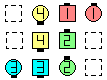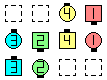\$B\$NA0(B \$B8e(B

\$B>e\$NNc\$G(B, 2 \$B\$D\$N(B 8-spot formation \$B\$O(B: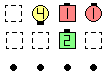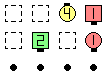\$B>e\$N(B 2 x 4 before \$B>e\$N(B 2 x 4 after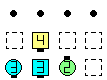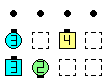\$B2<\$N(B 2 x 4 before \$B2<\$N(B 2 x 4 after
Triple Lines Working Backward Vertical Tag The Line: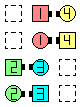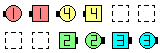\$B\$NA0(B \$B8e(B

\$B>e\$NNc\$G(B, 2 \$B\$D\$N(B 8-spot formation \$B\$O(B: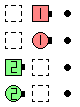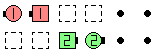\$B:8\$N(B 2 x 4 before \$B:8\$N(B 2 x 4 after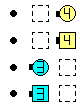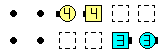\$B1&\$N(B 2 x 4 before \$B1&\$N(B 2 x 4 after
Triple Boxes Working Left Regroup: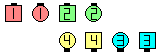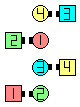Triple BoxesWorking Left Regroup\$B\$NA0(B \$B8e(B